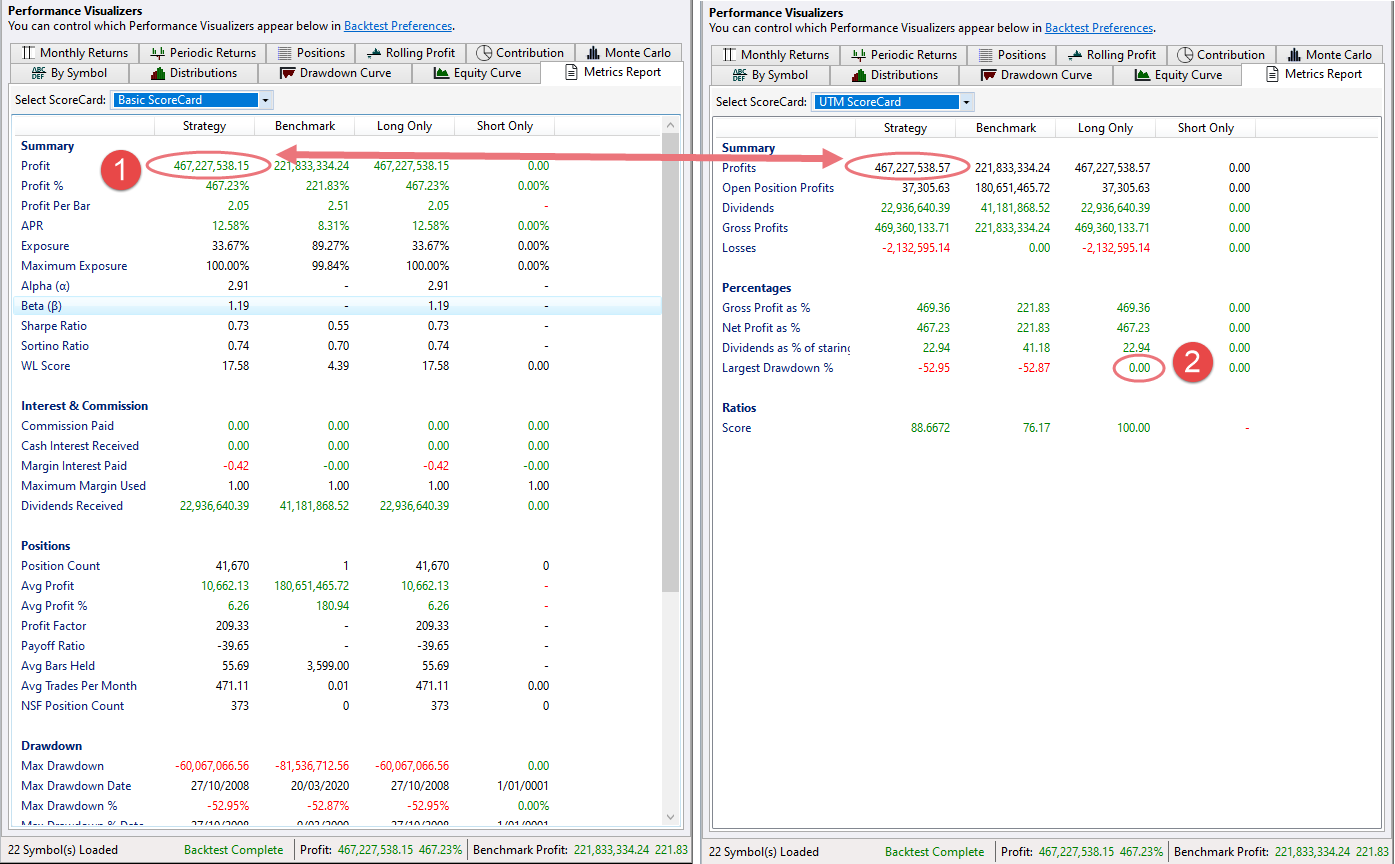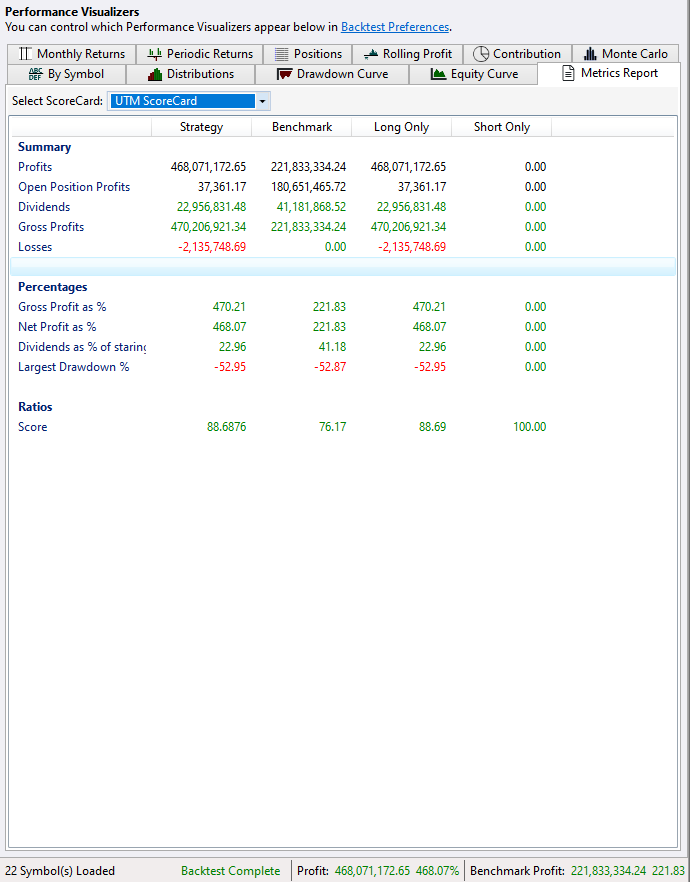I'm on a steep learning curve with the WL7 Extensions (which are awaesome). Is there a github repository or online source of complete examples that I can learn from?

The https://www.wealth-lab.com/Support/ExtensionApi pages have complete examples for some but not all extensions.

If there is no repository as yet, perhaps we (the community) could start creating one with a new "Example Code" tag for forum posts?
2
146

Bookmark

Sort
I''ve managed to get a Scorecard working but I have a couple of issues i can't seem to resolve.

1) For some reason I keep getting a different value (by about \$0.40) for Profit compared with the Basic ScoreCard.

Whats more perplexing is that the calculation on the benchmark is producing the same value as the Basic ScoreCard even though thesame code runs for both strategy and benchmark.

2) The Drawdown % is calculated for the Strategy column, but not for the "Long Only" column.

Code (vb.net) and Image below with 1 & 2 respectively.

Can anyone shed some light on this? It would save me some hair!```CODE:        Public Overrides Sub Initialize(ByVal bt As Backtester)

Dim Profit As Decimal = 0
For Each pos As Position In bt.Positions
If pos.Profit > 0 Then Profit += pos.Profit
Next

Dim OpenPositionProfit As Decimal
For Each pos As Position In bt.OpenPositions
If pos.Profit > 0 Then OpenPositionProfit += pos.Profit
Next

Dim Loss As Decimal = 0
For Each pos As Position In bt.Positions
If pos.Profit < 0 Then Loss += pos.Profit
Next

Dim LargestDrawdownPct As Decimal = 0.0
For i = 0 To bt.DrawdownPctCurve.Count - 1
If bt.DrawdownPctCurve.Item(i) < LargestDrawdownPct Then LargestDrawdownPct = bt.DrawdownPctCurve.Item(i)
Next

bt.Metrics.MyGrossProfits = CDbl(Profit + bt.DividendsPaid)
bt.Metrics.MyProfits = CDbl(Profit + bt.DividendsPaid + Loss)
bt.Metrics.MyOpenPositionProfits = CDbl(OpenPositionProfit)

bt.Metrics.MyDividends = bt.DividendsPaid
bt.Metrics.MyLosses = CDbl(Loss)
bt.Metrics.MyNetProfitPct = bt.NetProfitPct

Dim GrossProfitPct As Decimal = (((Profit + bt.DividendsPaid) / bt.StartingCapital) * 100)
bt.Metrics.MyGrossProfitPct = CDbl(GrossProfitPct)

bt.Metrics.MyDividendsAsPctCapital = CDbl((bt.DividendsPaid / bt.StartingCapital) * 100)
bt.Metrics.MyLargestDrawdownPct = CDbl(LargestDrawdownPct)

bt.Metrics.MyRatio = CDbl(100 - (Math.Abs(LargestDrawdownPct / bt.NetProfitPct) * 100))

End Sub```

0
Give this a try for drawdown:
`CODE:bt.Metrics.MyLargestDrawdownPct = bt.EquityCurve.MaxDrawDown`
0
Thanks Eugene. That fixed the Long Only column data popultion.

```CODE:bt.metrics.MyLargestDrawdownPct = bt.EquityCurve.MaxDrawDown '\$ value of drawdown.
bt.metrics.MyLargestDrawdownPct = bt.EquityCurve.MaxDrawDownPct ' % value of drawdown.```0
Mm, I think it's time to make my own scorecard as I'm already tired of scrolling and skipping metrics I never use).
0
Wait a week, there is help on the way...
2